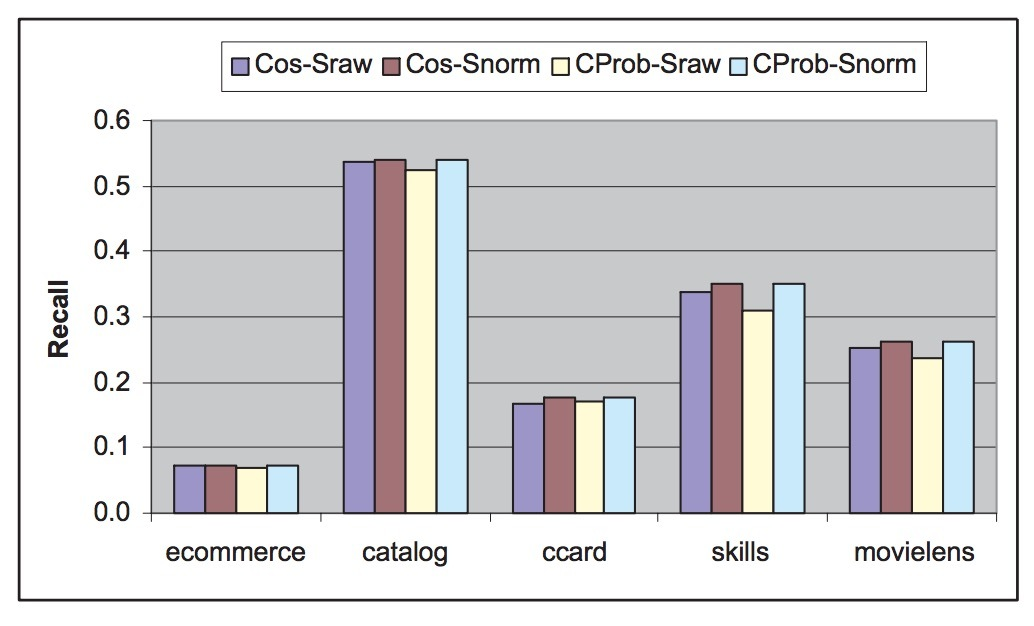# mllib中lda源码分析(二)

mllib中lda源码分析(一)中，我们描述了LDA的原理、以及变分推断batch算法和online算法的推导。这一节会再描述下spark MLlib中的ml实现。

spark MLlib的实现，是基于变分推断算法实现的，后续的版本会增加Gibbs sampling。本文主要关注online版本的lda方法。(代码主要以1.6.1为主)

# 一、Batch算法# 二、Online算法ml中的lda相当于一层wrapper，更好地适配ml中的架构(tranformer/pipeline等等)，调用的实际上是mllib中的lda实现。

## variationalTopicInference

ok。关于alpha的更新等，此处不再解释。详见源码。

# 介绍

• 一个词(word)是离散数据的基本单位，被定义成词汇表中的一个item，由{1,…,V}进行索引。我们将词表示成one-hot格式。使用上标来表示components，在词汇表中第v个词被表示成一个V维的向量w，其中$w^v=1, w^u=0(u\neq{v})$。
• 一个文档(document)是一个N个词组成的序列，表示成W=(w1,w2,…,wN)，其中wn是序列上的第n个词。
• 一个语料(corpus)是一个关于M个文档的集合，被表示成D={W1,W2,…,Wm}.

(blei论文中用黑体的w表示文档，为便于区分，此处用大写W)。

# LDA

LDA是一个关于语料的生成概率模型。基本思想是，文档可以被表示成在隐主题(latent topics)上的随机混合，其中每个主题(topic)都以一个在词(words)上的分布进行表示。

• 1.选择 N ~ Poisson(ξ)
• 2.选择 θ ~ Dir(α)
• 3.对于每个文档（N个词汇wn）:
• (a) 选择一个主题$z_n$ ~ Multinomial(θ)
• (b) 以主题$z_n$为条件，使用多项分布条件概率（multinomial probability）：$P(w_n \mid z_n, \beta)$选中一个词$w_n$

• 首先，Dirichlet分布的维度k(主题变量z的维度)，假设是已知并且确定的。
• 第二，词概率由一个k x V的矩阵β进行参数化，其中$\beta_{ij}=p(w^j=1 \mid z^i=1)$, 目前当成是一个待估计的确定量。
• 最后，Poisson猜想是不严格的，可以使用更多的实际文档长度分布。注意N对于所有其它数据生成变量(θ和z)是独立的。它是个辅助变量，我们通常忽略它的随机性。

$p(\theta|\alpha)=\frac{\Gamma(\sum_{i=1}^{k}\alpha_{i})}{\prod_{i=1}^{k}\Gamma(\alpha_{i})}{\theta_{1}}^{\alpha_{1}-1}...{\theta_{k}}^{\alpha_{k}-1}$ ……(1)

$p(\theta,z,W|\alpha,\beta)=p(\theta|\alpha) \prod_{n=1}^{N}p(z_n|\theta)p(w_n|z_n,\beta)$ ……(2)

$p(W|\alpha,\beta)=\int p(\theta|\alpha)(\prod_{n=1}^{N}\sum_{z_n}p(z_n|\theta)p(w_n|z_n,\beta))d\theta$ ……(3)

$p(D|\alpha,\beta)=\prod_{d=1}^{M}\int p(\theta_{d}|\alpha)(\prod_{n=1}^{N}\sum_{z_{dn}}p(z_n|\theta)p(w_n|z_n,\beta))d\theta_{d}$

LDA可以表示成图1所示的概率图模型。有三个级别的LDA表述。

• 语料级参数：α 和 β是语料级参数（corpus-level parameters），假设在生成一个语料的过程中只抽样一次。
• 文档级变量：θd是文档级变量（document-level variable），每个文档抽样一次。
• 词级别变量：$z_{dn}$和$w_{dn}$是词级别变量（word-level variables），对于每个文档中的每个词抽样一次。## 2.1 LDA与可交换性

$p(z_1,...,z_N)=p(z_{\pi(1)},...,z_{\pi(N)})$

De Finetti’s representation理论声称：一个无限可交换的随机变量序列的联合概率，如果一个随机参数从这些分布中抽取，那么问题中的随机变量，在其于该参数条件下是独立同分布的（iid: independent and identically distributed）。

$p(W,z)=\int p(\theta) (\prod_{n=1}^{N} p(z_n|\theta) p(w_n|z_n)) d\theta$

## 2.2 其它模型

### unigram模型

$p(W)=\prod_{n=1}^{N} p(w_n)$

### Mixture of unigrams

$p(W)=\sum_{z} p(z) \prod_{n=1}^{N} p(w_n|z)$

### pLSI

pLSI是另一个广泛使用的文档模型(Hofmann 1999)。pLSI模型认为，一个文档d，词wn，对于一个未观察到的主题z来说是条件独立的：

$p(d,w_n)=p(d)\sum_z p(w_n|z) p(z|d)$

pLSI模型尝试放宽maxture of unigrams模型中作出的简化猜想：每个文档只由一个主题生成。在某种意义上，它会捕获概率：一个文档可以包含多个主题，因为p(z | d)可看成是对于一个特定文档d的主题权重的混合。然后，注意，d是一个dummy index，指向在训练集中文档列表。这样，d是一个多项分布随机变量，值尽可能与训练文档一样多，模型学到的主题混合 p(z | d)只对应于被训练的文档。出于该原因，pLSI并不是一种定义良好的（well-defined）文档生成模型；天然不支持使用它来分配概率给一个之前未见过的文档。## 2.3 几何学解释

LDA与其它隐主题模型不同，可以通过隐空间(latent space)的几何表示来解释。来看下在每种模型下，一个文档在几何上是如何表示的。

unigram模型是在word simplex上找到单个点，假定语料中的所有词都来自该分布。隐变量模型（letent variable models）会考虑在word simplex上的k个点，并基于这些点形成一个子simplex(sub-simplex)，我们称之为topic simplex。注意，在topic simplex上的任意点，在word simplex也是一个点。使用topic simplex的不同隐变量模型，以不同的方式生成一个文档。

• 1-gram混合模型（mixture of unigram model）：假设对于每个文档，在word simplex上的k个点（也就是说：topic simplex的其中一个角落）被随机选中，该文档的所有词汇都从这些点的分布上抽取得到。
• pLSI模型：假定一个训练文档的每个词都来自一个随机选中的topic。这些主题(topics)本身从一个在这些主题上的指定文档分布上抽取到，例如，在topic simplex上的一个点。对于每个文档都有一个这样的分布；训练文档的集合定义了在topic simplex上的一个经验分布。
• LDA: 假定，已观察到和未观察到的文档上的每个词，都由一个随机选中的topic生成，这个topic从一个随机选中的参数的分布上抽取。该参数从来自topic simplex的一个平滑分布上的每个文档中抽样得到。# 3.推断与参与估计

## 3.1 inference

$p(\theta,z|W,\alpha,\beta)=\frac{p(\theta,z,W|\alpha,\beta)}{p(W|\alpha,\beta)}$

$p(W|\alpha,\beta)=\frac{\Gamma(\sum_i\alpha_i)}{\prod_{i}\Gamma(\alpha_i)} \int (\prod_{i=1}^{k}\theta_{i}^{\alpha_i-1}) (\prod_{n=1}^{N}\sum_{i=1}^{k}\prod_{j=1}^{V}(\theta_i\beta_{ij})^{w_n^j}) d\theta$

## 3.2 变分推断

$q(\theta,z|\gamma,\phi)=q(\theta|\gamma)\prod_{n=1}^{N}q(z_n|\phi_n)$ ……(4)$(\gamma^{*},\phi^{*})=arg min_{\gamma,\phi}^{} D(q(\theta,z|\gamma,\phi)||p(\theta,z|W,\alpha,\beta))$……(5)

$\phi_{ni} \propto \beta_{iw_n}exp\{E_q[log(\theta_i)|\gamma]\}$……(6)

$\gamma_{i}=\alpha_{i}+\sum_{n=1}^N\phi_{ni}$ ……(7)

$E_q[log(\theta_i)|\gamma]=\Phi(\gamma_i)-\Phi(\sum_{j=1}^{k}\gamma_{j})$ ……(8)## 3.3 参数估计

$l(\alpha,\beta)=\sum_{d=1}^{M}logp(W_d|\alpha,\beta)$

• 1.(E-step): 对于每个文档，找到变分参数{$\gamma_d^{\ast},\phi_d^{\ast}: d \in D$}。这个在上一节已描述。
• 2.(M-step): 分别根据模型参数α 和 β，最大化在log likelihood上产生的下界。这相当于，在E-step中计算出的合适的后验概率下，为每个文档使用期望的足够统计量，找到极大似然估计。

$\beta_{ij} \propto \sum_{d=1}^{M}\sum_{n=1}^{N_d}\phi_{dni}^{*}w_{dn}^j$ ……(9)

## 3.4 Smoothing$q(\beta_{1:k},z_{1:M},\theta_{1:M}|\lambda,\phi,\gamma)=\prod_{i=1}^{k}Dir(\beta_i|\lambda_i) \prod_{d=1}^M q_d(\theta_d,z_d|\phi_d,\gamma_d)$

$\lambda_{ij}=\eta + \sum_{d=1}^{M} \sum_{n=1}^{N_d} \phi_{dni}^{*} w_{dn}^j$

# 4.online算法

• 首先，从主题$\theta_d \sim Dirichlet(\alpha)$上抽取一个分布；
• 接着，对于在文档中的每个词i，从主题权重$z_{di} \sim \theta_d$ 上抽取一个主题索引$z_{di} \in {1, . . . , K }$，从选中的主题上抽取观察词$w_{di}$，$w_{di} \sim \beta_{z_{di}}$。出于简洁性，我们假设在θ 和 β上具有对称先验，但该假设很容易放宽。

• 主题(the topics): β
• 主题比例(topic proportions): θ
• 主题分配(topic assignments): z

## 4.1 Batch算法

$logp(W|\alpha,\eta) \geq \mathcal{L} (W,\phi,\gamma,\lambda) \triangleq E_q[logp(W,z,\theta,\beta|\alpha,\eta)] - E_q[logq(z,\theta,\beta)]$ …… (1)

$q(z_{di}=k)=\phi_{dw_{di}k}$ $q(\theta_d)=Dirichlet(\theta_d;\gamma_d)$ $q(\beta_k)=Dirichlet(\beta_k;\lambda_k)$

……(2)

$\mathcal{L} (W,\phi,\gamma,\lambda) = \sum_{d} \{ E_q[log p(w_d | \theta_d,z_d,\beta)] \\ + E_q [log p(z_d | \theta_d)] - E [logq(z_d)] + E [logp(\theta_d|\alpha)] - E [log q(\theta_d)] \\ + ( E [logp(\beta |\eta)] - E [log q(\beta)])/D \}$

……(3)

$\mathcal{L} = \sum_d \sum_w n_{dw} \sum_k \phi_{dwk}( E_q[log\theta_{dk}] + E[log\beta_{kw}] - log\phi_{dwk}) \\ - log\Gamma(\sum_k\gamma_{dk}) + \sum_k(\alpha-\gamma_{dk})E[log\theta_{dk}] + log\Gamma(\gamma_{dk}) \\+ (\sum_k -log\Gamma(\sum_w\lambda_{kw}) + \sum_w(\eta-\lambda_{kw})E[log\beta_{kw}] + log\Gamma(\lambda_{kw}))/D \\ + log\Gamma(K\alpha) - K log\Gamma(\alpha) + (log\Gamma(\mathcal{V}\eta)-\mathcal{V}log\Gamma(\eta))/D \\ \triangleq \sum_d \ell(n_d,\phi_d,\gamma_d,\lambda)$

……(4)

L可以使用在变分参数φ, γ, λ 上的坐标上升法进行优化：

$\phi_{dwk} \propto exp \{ E_q[log\theta_{dk}] + E_q[log\beta_{kw}]\}$ $\gamma_{dk}=\alpha + \sum_{w}n_{dw}\phi_{dwk}$ $\lambda_{kw}=\eta+\sum_d n_{dw} \phi_{dwk}$

……(5)

$E[log\theta_{dk}]=\Phi(\gamma_{dk})- \Phi(\sum_{i=1}^K \gamma_{di})$ $E[log\beta_{kw}]=\Phi(\gamma_{kw})- \Phi(\sum_{i=1}^V \gamma_{ki})$

……(6)## Online算法

$\mathcal{L}(n,\lambda) \triangleq \sum_d \ell (n_d,\gamma(n_d,\lambda), \phi(n_d,\lambda), \lambda)$ ……(7)

$\ell(n_d,\gamma_d,\phi_d,\lambda)$是第d个文档对等式(4)的变分上界的贡献度。这类似于最小平方矩阵分解的目标函数，尽管LDA的ELBO比简单的squared loss function更不方便。

Online VB for LDA在算法2中描述。Mini-batches: 在随机学习中的常用技术是，在每次更新时考虑多个观察（observations）以降噪。在online LDA中，这意味着计算$\hat{\lambda}$使用S>1的观察：

$\hat{\lambda}_{kw}= \eta + \frac{D}{S} \sum_{S} n_{tsk} \phi_{tskw}$ ……(8)

$\alpha \leftarrow \alpha - \rho_{t} \hat{\alpha}(\gamma_t)$ $\eta \leftarrow \rho_{t} \hat{\eta}(\lambda)$

……(9)

# 介绍

MLlib中有两个lr实现。分别在mllib和ml中。此处分析的是ml，这也是后续mllib继续重点发展的一个库, mllib则会渐渐淡出历史舞台。ml的logistic回归，代码在org.apache.spark.ml.classification.LogisticRegression中实现。在详见代码之前，我们先简单地看下LR可调节的参数. [暂时修正至以1.6.1代码为参考.]

# 一、LR模型的参数

• threshold: 如果label 1的概率>threshold，则预测为1，否则为0.
• regParam：正则项参数(相当于 $\lambda$)
• elasticNetParam：ElasticNet混合参数 $\alpha$ 。如果$\alpha = 0$, 惩罚项是一个L2 penalty。如果$\alpha = 1$，它是一个L1 penalty。如果$0 < \alpha < 1$，则是L1和L2的结果。缺省为0.0，为L2罚项。
• maxIter: 缺省100次。
• tol：迭代收敛的容忍度。值越小，会得到更高精度，同时迭代次数开销也大。缺省为1E-6.
• fitIntercept: 是否要拟合截距项(intercept term)，缺省为true.
• standardization：在对模型进行fit前，是否对训练特征进行标准化(归一化)。模型的系数将总是返回到原比例(origin scale)上，它对用户是透明的。注意：当不使用正则化时，使用/不使用standardization，模型都应收敛到相同的解（solution）上。在R的GLMNET包里，缺省行为也为true。缺省为true。
• weightCol：如果没设置或空，所有实例为有为1的权重。如果设置，则相当于对unbalanced data设置不同的权重。缺省不设置。
• treeAggregate：如果特征维度或分区数太大，该参数需要调得更大。缺省为2.

# 二、原理

logistic回归的原理，这里不详述。简单地写：y = logit(∑wx + b)。相应的loss和梯度如下所示（箭头表示向量）：

$l_{total}(\vec{w}, \vec{x})=l_{model}(\vec{w}, \vec{x})+l_{reg}(\vec{w})$ $\vec{G} (\vec{w}, \vec{x})_{total}= \vec{G} (\vec{w}, \vec{x})_{model} + \vec{G} (\vec{w})_{reg}$

$l_{model}$为cross-entropy；$l_{reg}$为正则项。

$(\vec{G}(\vec{w}, \vec{x})_{model})_i= G_i(\vec{w}, \vec{x})= \frac{\partial{l(\vec{w}, \vec{x})}}{\partial{w_i}}=\sum_{k=1}^{N}y_{k} x_{ki} - \frac{exp(\vec{x}_{k} \vec{w})}{1+exp(\vec{x}_k,\vec{w})}x_{ki}$

$l(\vec{w}, \vec{x})_{model}=-\sum_{k=1}^{N}y_{k} \vec{x}_{k} \vec{w} - log(1+exp(\vec{x}_k \vec{w}))$# 四、正则

• L2 regularization -> ridge
• L1 regularization -> lasso
• mix L1 and L2 -> elastic Net

$l_{reg}(\vec{w})=\lambda\sum_{i=1}^{N}{w_{i}}^2$ $l_{reg}(\vec{w})=\lambda\sum_{i=1}^{N}|w_i|$ $l_{reg}(\vec{w})=\lambda\sum_{i=1}^{N}(\frac{\alpha}{2}{w_i}^2+(1-\alpha)|w_i|)$

## LBFGS和OWLQN

ok，我们知道，模型本身基本上核心代码就落在了这两个方法上：LBFGS和OWLQN。两者都是牛顿法的变种，核心思想是：

$\vec{w}_{n+1}=\vec{w_n}-H^{-1}\vec{G}$

L-BFGS: Limited-memory BFGS。其中BFGS代表4个人的名字：broyden–fletcher–goldfarb–shanno OWL-QN: (Orthant-Wise Limited-Memory Quasi-Newton)算法。

breeze库用于数值处理。它的目标是通用、简洁、强大，不需要牺牲太多性能。当前版本0.12。我们所熟悉的spark中的MLlib（经常见到的线性算法库：breeze.linalg、最优化算法库：breeze.optimize）就是在它的基础上构建的。另外它还提供了图绘制的功能（breeze.plot）。

# 总结

• 缺省会对特征做归一化，对于一些场景（比如推荐），离散化的特征，归一化没啥意义。反倒可能会影响结果好坏。
• 对于截距b，会使用正负样本比例，进行log(P(1)/P(0))初始化。收敛会更快。
• cache机制(StorageLevel.MEMORY_AND_DISK)。当内存不够用，可能会影响性能，这一点不好。

# 介绍

CF的最流行方法是itemCF。它可以提供高质量的top-k结果。它的主要思想是：计算一个similarity matrix，相应的entries对应于pairwise item相似度（Person相关系数是一种流行选择）。为active users从头到尾计算推荐开销很大，因此，CF-based推荐通常会预计算好item-wise相似度，并以一种可快速检索的方式存储它们。根据该方法，预测active user ($u_i$)在item ($v_j$)上的得分的任务包含了以下steps：

• 1.为$v_j$寻找N个最相似的items（被$u_i$评分过），这被称为$v_j$在$u_i$ profile中的N个最近邻。
• 2.基于在这N个items上的用户评分，通过相似度计算一个加权平均

• (1) 我们需要处理：每个candidate item的聚合得分必须通过来自一个不同的lists集合(set)的entries来决定。这是因为：在一个给定user profile中，每个candidate item的N个最相似items可能不同
• (2) 加权平均并不是一个单调聚合函数（monotone aggregate function），该特性会在经典top-k算法中常使用. 在top-k算法中有一些工作是使用非单调聚合函数的[10,18,21]，据我们所知，在top-k问题上还没有工作是使用非单调聚合函数来解决lists的多个集合的组合。

• (i) 数据层（Data layer）: 包含了user/item rating matrix
• (ii) 中间层（Intermediate layer）: 由item-item matrix组成，需要让similarity matrix时刻保持最新
• (iii) 应用层（Application layer）: 在中间层数据之上运行的高效运行topk算法。该层会使用相似度阀值(通过cost-based最优化得到)来调用probe操作，并通过explore操作寻找top-k itemstop-k算法可以独立于中间层（intermediate layer），并可以访问中间层最新的数据结构。我们提出了一个two-phase top-k算法。在第一阶段，我们会基于相似度值计算一个阀值。该阀值以这样的方式进行寻找：最大化（最小化）相似度值更大（更小）的items的概率，接着该阀值会介于items的N个最近邻之间。在第一phase后，相似度矩阵的大部分会被过滤，size问题会极大缩减。第二个phase会使用剩下的值来计算要预测的ratings。我们会定义一个目标函数，它是两个phases的期望cost的一个上限，会寻找关于threhold的最优值来最小化该cost。我们会使用概率分布来建模相似度矩阵，以便进行概率分析。我们提出了一种高效算法来寻找top-k推荐。我们的贡献如下：

• 1.为itemCF提出了一种分层系统。
• 2.提出了两种naive算法，作为baseline来校准算法效果。
• 3.我们展示了基于TA/NRA算法扩展的方法
• 4.为itemCF设计了一种新的top-k算法
• 5.根据运行时间和top-k结果质量来评估算法

# 2.相关工作

ItemCF和可扩展性：User-CF算法会基于N个最近邻用户(对该item评过分)来预测未知评分. 它需要计算和维护一个n x n的user-user相似度矩阵。用户数n通常大于items数m。Item-CF可以克服这个缺限。除了对可扩展性改进外，它会寻找pairwise相似度来产生更好的accuracy。在ItemCF中，一个active user在一个candidate item上的rating会基于与该用户在该candidate item相似的N个items上的rating进行预测。

top-K算法：topK算法被广泛研究。大多数相关工作构建在经典的TA/NRA算法之上。这些算法假设有一个单调聚合函数，以及一个关于多个列表的集合，在这之上执行聚合是事先知道的。对比这些算法，在我们的问题中，面临着两个主要挑战：

• i) 我们的聚合函数不是单调的(monotone)
• ii) 列表集合中被聚合的值是不固定的，可能会随着item间相互变化

# 3.先决条件与Naive算法

• R：稀疏的$n \times m$矩阵
• $r_{ij}$：为entry（表示在第i个user上对第j个item的评分(rating)），值采用$\lbrace 1, 2, \cdots, C \rbrace, C>1$来表示

• $u_i$：表示第i个user(row)。注意，从行为角度看，我们可以使用row $u_i$表示第i个user（列也如此）
• $v_j$：表示第j个item(column)
• $r \in u_i$：表示一个在user $u_i$的row上的已存在评分（existing rating）
• $\hat{r}_{ij}$：来做为active user $u_i$在一个candidate item $v_j$上的未知评分(unknown rating)的预测值。
• $\mu_i$：作为被$u_i$评分过的items数目
• $\mu$：表示被任意user评分过的items平均数目

$\hat{r}_{ij} = (\sum\limits_{x=1}^N s_{xj} \times r_{ix}) / ( \sum\limits_{v_y \in N(v_j,u_i)} s_{yj})$

…(1)

## 3.1 Naive-1算法

• 1.使用等式(1)为每个单独的candidate item $v_j$预测score

• 2.一旦每个item的score在前一步被计算，寻找具有最高scores的k个items的时间复杂度为$O(m logk)$

• (i) 探测(Probe)：探测每个candidate item，以便寻找在user profile中的最相似的N个items。
• (ii) 探索(Explore)：探索关于candidate items的list，并计算它们的scores，接着寻找具有最高scores的top-k items。

step (ii)是必要的，以便寻找到具有最好得分的k个items。对于任意item，决定它的score需要我们知道与它最相似的N个items（由step(i)得到）。

probe阶段涉及到$O(m\mu_i logN)$个操作，而explore阶段需要$O(m(logk + N)))$个操作。实际上，N的值通常为30或更小。另外，logk通常不超过5, 主要原因是我们不希望用户被太多的推荐所淹没。在probe开销中$\mu_i$因子的存在，表明：Naive1算法的probe部分的开销占支配地位。例如，在MovieLens数据集中，有100w个ratings，$\mu_i$的平均值为165. 而在Netflix数据集中，100M的ratings、500k users，每个user的ratings平均数目为200.

## 3.2 Naive-2算法$L_i \in L$表示与item $v_i$相关的list。

• $L_i$的elements：是形如(item_pointer, similarity)的pairs
• lists以非增的相似度(与$v_i$)进行排序；
• item_pointer：是一个指向item所在的实际内存位置的指针，或者简化为item id。所使用的pointers在ratings matrix R以及相似列表L间的具有一个统一的items表示。
• $L_{ij}$：表示list $L_i$的第i个entry。$L_{ij}$的similarity表示对于$v_i$的第j个最相似item。

• 1.标记由$u_i$评分的所有$\mu_i$个items，时间复杂度为$O(\mu_i)$
• 2.对于每个candidate item $v_j$，从该list的头部头取$L_j$，直到发现N个已标记items。
• 3.计算聚合得分，并发现具有最高得分的k个items，时间复杂度为$O(m log k)$

。。。

# 4.经典的top-k算法

• (i) 这种算法可以以有序方式访问L的列，对应于$u_i$评过分的items
• (ii) 这种算法可以访问L的列，对应于candidate items

# 5. two-phase算法

## 5.1 two-phase算法

$LP_{ij}.prob$值可以通过对具有一个合适分布的similarity matrix建模来估计，后续会介绍。当我们使用一个分布来建模similarity matrix时，不需要提供prob values。作为替代，threshold可以被直接转化成一个相似度threshold。出于简洁性，我们假设给定这样的概率。注意：LP的elements包含的similarity值越高，prob值越低。因此，保持LP的列以similarities的非递增顺序进行排序，等同于保持以prob值的非递减顺序进行排序。

# 3

## 3.1 相似度选择

• cosine-based
• Conditional Probality-based

# 4.实验

## 4.3 相似度归一化的效果